### The Birthday Problem

September 25, 2023

Prior to starting a mathematical mathematical proof, it's important to check the veracity of your axioms. Axioms are concepts that are so evident that they are accepted without question. Geometrical axioms were called postulates in Euclid's Elements, and postulates in The Elements are such things as the ability to draw a straight line between any two points. Euclid's fifth postulate, also known as the parallel postulate, admits the possibility of parallel, non-intersecting lines. While this is true for the two-dimensional space of Euclidian geometry, it isn't true for curved surfaces such as the surface of a sphere. For that reason, a triangle drawn on the surface of a sphere has a sum of interior angles that's greater than 180 degrees.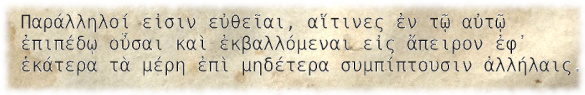Euclid's fifth postulate, the parallel postulate, Book 1, Definition 23, of his Elements.

The English translation reads, "Parallel lines are straight lines which, being in the same plane, and being produced to infinity in each direction, meet with one another in neither (of these directions)."

Greek text and English translation from ref. 1. The background is derived from the Wikimedia Commons image of an early 9th century manuscript.

I was reminded of the importance of axioms by an experience that one of my grandsons had at a birthday party. Among the party treats were bags of M&M's candies. These chocolate candies have a thin coating colored red, orange, yellow, green, blue, and brown. He found that there were ten yellow pieces in one of his M&M's bags having twelve pieces.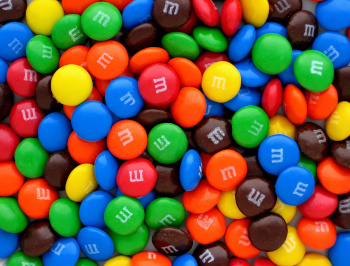If our axiom is that there's a uniform distribution of colors manufactured, the probability of getting at least ten yellow candies would be (1/6)10 = 1.65382x10-8, and the probability of getting exactly ten yellow candies would be (1/6)10·(5/6)2 = 1.14848x10-8.

While it's known that the color distribution deviates somewhat from uniformity, there's obviously something about the manufacturing process, such as non-uniform mixing, that's happened.

Wikimedia Commons image by Evan-Amos of the Vanamo Online Game Museum

Also related to birthdays is a probability problem known as the birthday problem. This problem is to determine the probability that at least two people in a group of N people will share a birthday. This is different from the probability that another person in the group will share your birthday, which is simply N/365, if we exclude leap year birthdays. Relaxing the requirement of matching a specific date to the condition that any two people will have matching birthdays gives the interesting result that N = 23 gives better than a 50:50 chance for a match. This is a consequence of there being so many pairs, N x (N-1)/2 pairs, which is 253 for N = 23 people.

There's a simple permutation equation for calculating the probability for any N. However, there's much more fun in doing a Monte Carlo simulation. I generally use PHP for simple simulations such as this, and I've released the PHP source code in this zip file. The following graph shows the probability of a shared birthday among a group of N people. The calculated value at N =23 is 0.5087. The table shows the probability for various values of N.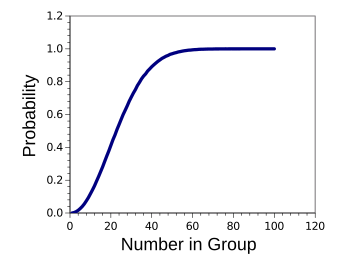Graph of birthday problem probabilities.

(Graphed using Gnumeric from data generated by my simulation program.

Birthday Problem Probabilities
(As found by a Monte Carlo simulation)

Number Probability       Number Probability
10 0.11714       60 0.99406
20 0.41197       70 0.99902
23 0.50868       80 0.99991
30 0.70617       90 0.99999
40 0.89099       100 1.00000
50 0.97015

The birthday problem has a practical use. In cryptanalysis, the birthday attack reduces the computation needed in finding hash collisions.

I decided to investigate another birthday problem, this one involving thermodynamics, rather than mathematics. This is the question of how long a birthday candle will burn. The first figure below shows two types of birthday candles, a smaller one for cupcakes, and a larger one for a large cake. The second figure shows the flame sizes of the two birthday candles, along with a dinner table candle for reference.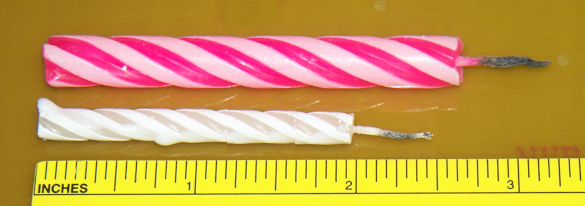Two sizes of birthday candles. The upper candle is a larger size for cakes, and the lower is a smaller size for cupcakes. (Photo by the author.)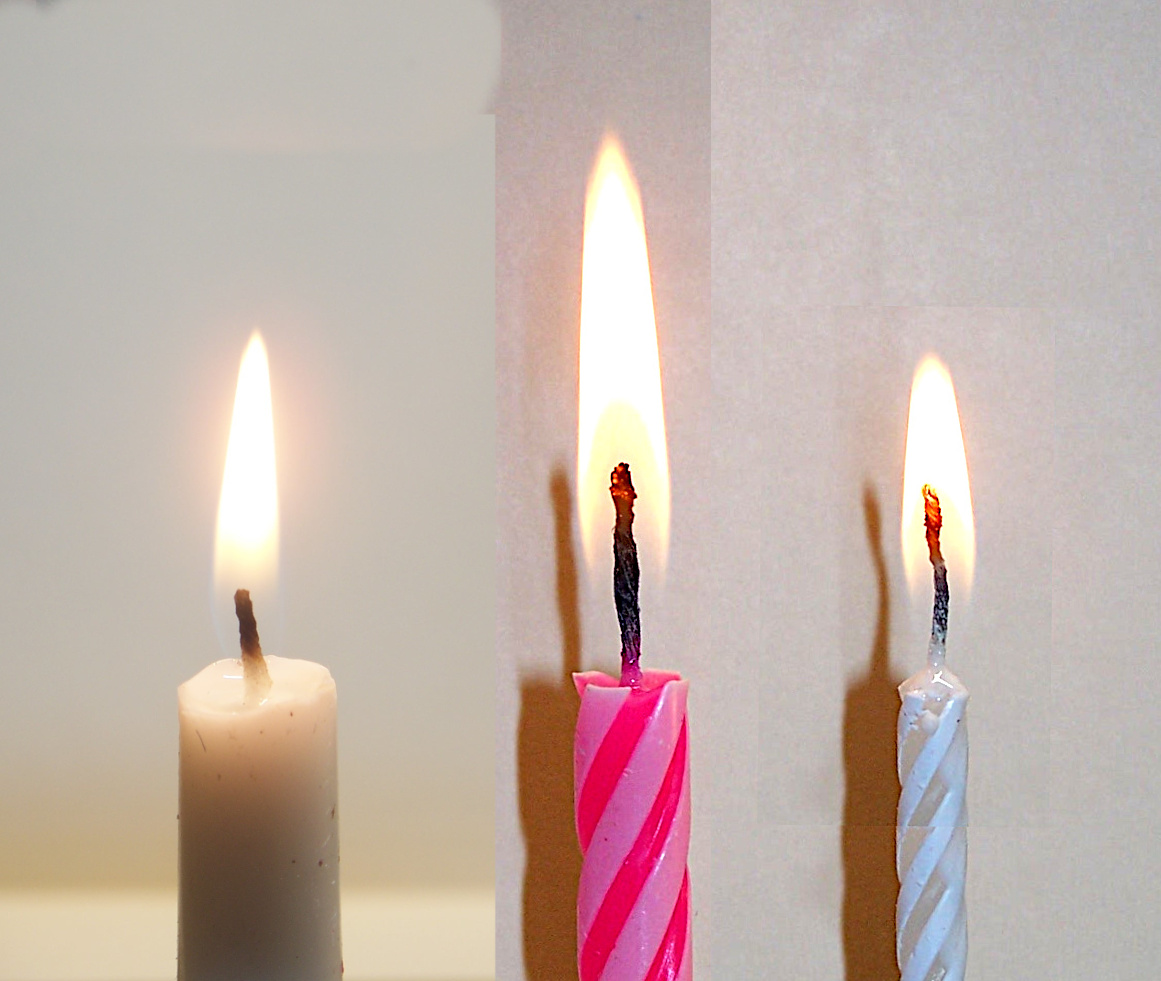A composite image of candle flames at the same scale. Leftmost is a large dinner table candle. Center is a large birthday candle for a cake. Right is a small birthday candle for a cupcake. Surprisingly, the flame size does not scale with candle size.

According to a study by the US National Institute of Standards and Technology (NIST), "the burning rate and heat flux from the candle flame depends on many interdependent factors including wick length, wick shape, mass burning rate, heat release rate, flame height, and paraffin wax formulation..."

(Photo by the author.)

Paraffin wax, a mixture of hydrocarbon molecules containing between 20 and 40 carbon atoms, is generally used as a candle material. The US National Institute of Standards and Technology (NIST) found that the heat of combustion (Hc) of a paraffin wax candle is about 43.8 kJ/g, and its heat release rate was 77 ± 9 W. My large birthday candle had a mass of 2.75 g, and my small birthday candle had a mass of 1.0 g. This leads to the lifetime calculation in the following table.

Quantity Small Candle Large Candle
Mass (g) 1.00 2.75
Hc(kJ/g) 43.8 43.8
Total Energy Released (J) 43,800 120,450
Energy Release Rate (W) 77 ± 9 77 ± 9